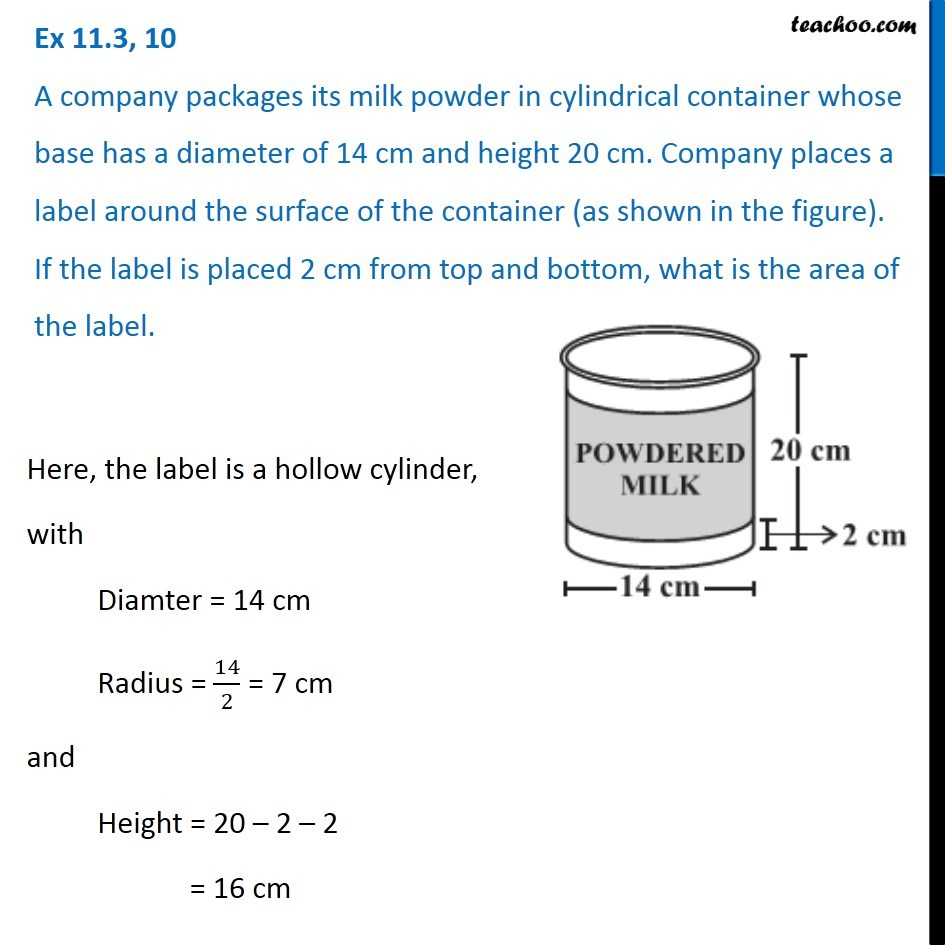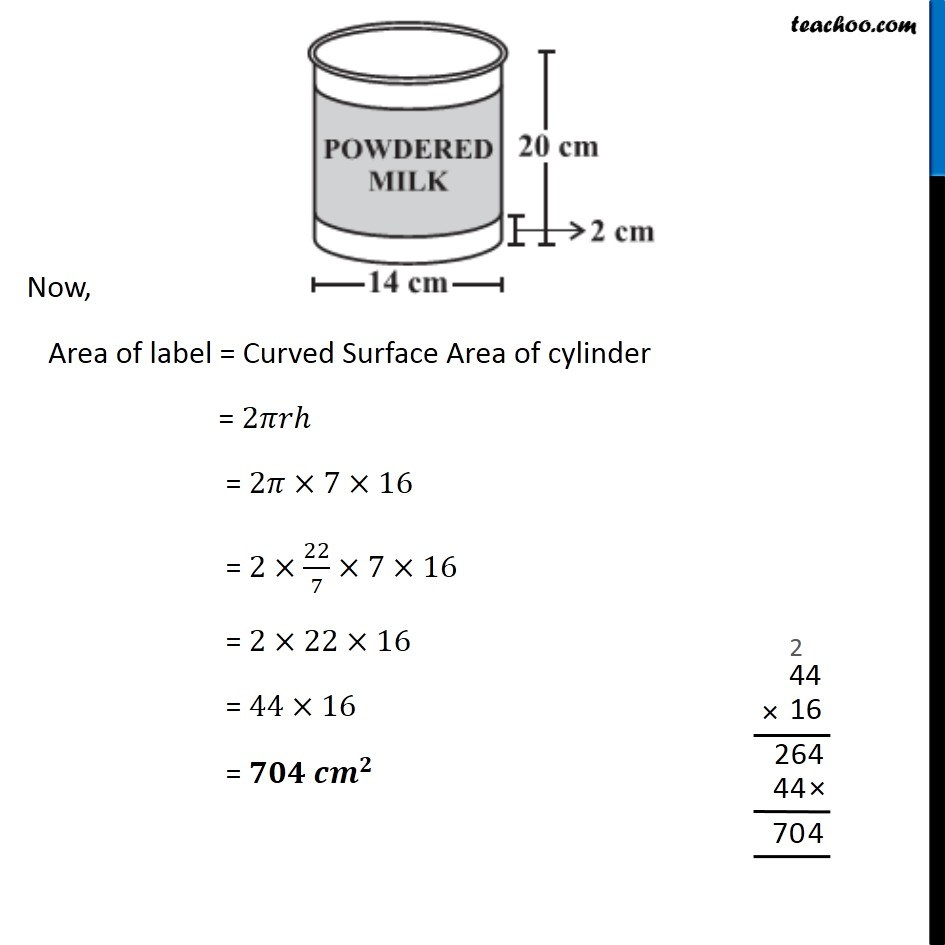Surface Area of Cylinder

Chapter 11 Class 8 Mensuration
Concept wiseIntroducing your new favourite teacher - Teachoo Black, at only ₹83 per month

### Transcript

Ex 11.3, 10 A company packages its milk powder in cylindrical container whose base has a diameter of 14 cm and height 20 cm. Company places a label around the surface of the container (as shown in the figure). If the label is placed 2 cm from top and bottom, what is the area of the label. Here, the label is a hollow cylinder, with Diamter = 14 cm Radius = 14/2 = 7 cm and Height = 20 – 2 – 2 = 16 cm Now, Area of label = Curved Surface Area of cylinder = 2𝜋𝑟ℎ = 2𝜋×7×16 = 2×22/7×7×16 = 2×22×16 = 44×16 = 𝟕𝟎𝟒 𝒄𝒎^𝟐